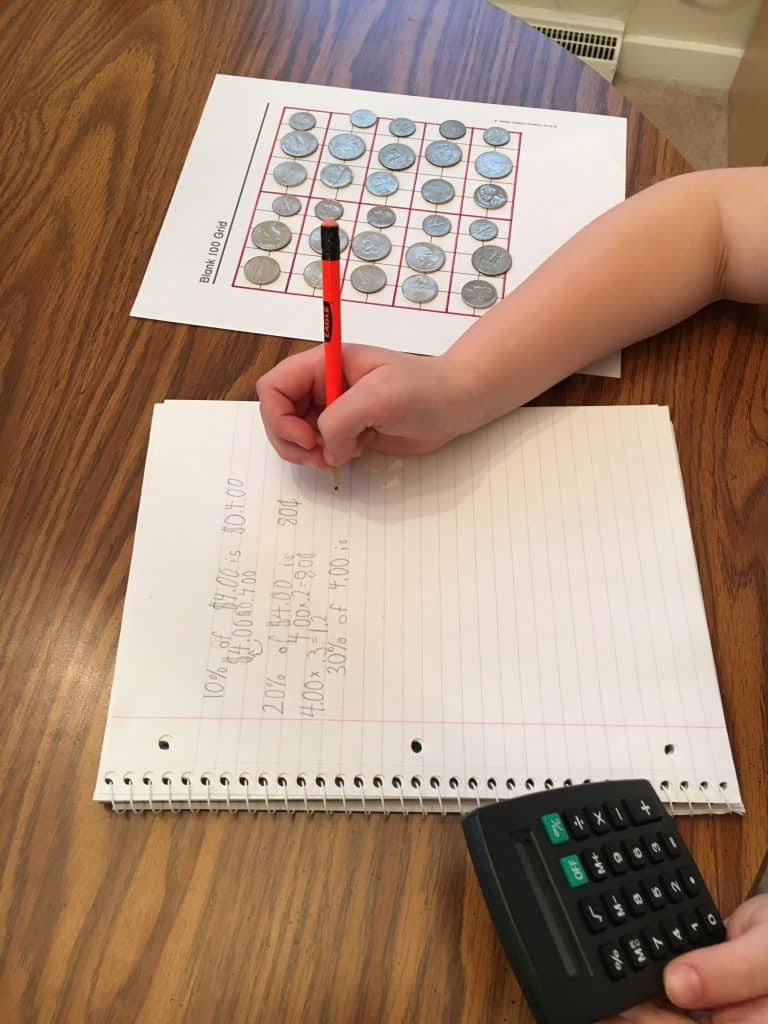Categories

# Practice Makes Perfect

Last month we started talking about percentages in the 10-10-80 savings plan with our focus on the 10% that goes to savings. I want J to save at least 10% of all money he receives, so we’re working on how to calculate 10% of any amount.

I showed him two ways to calculate 10% last time:

• Use the 100-grid and divide the amount into 10 equal parts. \$3.00 divided into 10 equal parts gives you 30 cents in each 10-block
• Move the decimal one place to the left to find 10%. \$3.00 becomes \$.300 or 30 cents

J got a calculator for Christmas, so we integrated it into our practice. I showed him how to find the decimal equivalent of a percentage by moving the decimal point two places to the left (10% becomes .10).

So we added another way to calculate 10%:

• Multiply the amount by .1. \$3.00 x .1 = .30 or 30 cents

The first time we practiced, he wrote out the question/problem and then the answer:

• Q: What is 10% of \$1.00?
• A: \$0.10

This time, he just wrote a complete sentence:

• 10% of \$4.00 is \$0.40We’ll be practicing again soon — stay tuned!